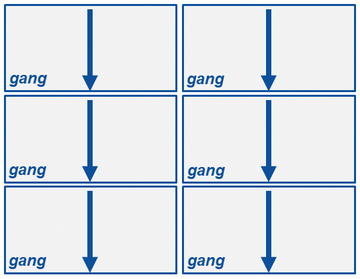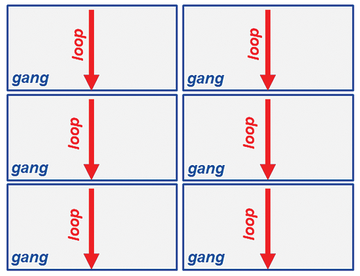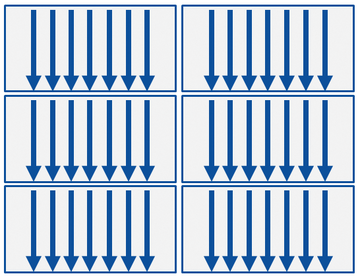# Parallelizing Code

#### Parallel Computing

Classically, applications were written to be executed serially. One computation is performed after another. But this approach doesn't take into account that some computations or regions can be computed simultaneously. Finding and parallelizing such regions of an application allows it to run faster and scale better than serial applications (see Amdahl's law ).

Today's processors have multiple cores, and accelerators such as GPUs have thousands of lightweight cores that can be used, as well. At a simplistic level, parallelization breaks a problem into discrete parts that can be solved simultaneously. Each part is then executed on different cores but with some sort of coordination.

One likely place for parallelization to occur is in loops. In this simple Fortran loop

```do i = 1,n
z(i) = x(i) * y(i)
enddo```

each value `z(i)` is not dependent on previous values of `z(i)`. Therefore, all values of `z(i)` can be computed in any order or at the same time. If the upper limit of the loop, `n`, is large enough, some processing hardware can greatly speed up the computation.

What happens if `z(i)` depends on a previous value, as in the following:

```do i = 2,n
z(i) = z(i-1)*2
enddo```

As written, you can't generally parallelize the loop because of data dependency . This dependency is also called loop-level parallelism. However, for this particular example, you could rewrite the loop in a way that can be parallelized:

```do i = 2,n
z(i) = z(i)*2**(i-1)
enddo```

When the compiler tells you a loop cannot be parallelized, you, the programmer, will need to determine whether it can be refactored in such a way that it can be parallelized.

In other situations, you might have to pay attention to race conditions, mutual exclusion, and parallel slowdown. For the purposes of this article, I won't cover these situations or conditions.

#### OpenACC Introduction

OpenACC has a number of directives that can be put into code that give the compiler information about how you would like to parallelize the code. The syntax's general form is shown in Table 1, but before getting too deep into directives, I'll look at what is happening in the code with the parallel directive.

Table 1

Parallelizing Fortran and C

Fortran C
`!\$acc <directive clauses>`` < code >` `:#pragma acc <directive clauses>`` < code >`

The `parallel` directive is a way to express parallelism in your code. Table 2 is a very simple example of getting started with directives in both Fortran and C.

Table 2

parallel Directive

Fortran C
`!\$acc parallel`` < parallel code >``!\$acc end parallel` `#pragma acc parallel``{`` < parallel code >``}`

The directives for Fortran and C begin with comment characters in their respective languages so that a non-OpenACC-compliant compiler will ignore them.

In Fortran, immediately after the comment (`!`) is `\$acc`, which informs the compiler that everything that follows is an OpenACC directive. In C, immediately after the comment (`#`) is `pragma`, informing the compiler that some sort of directive follows. After `pragma`, the `acc` tells the compiler that everything after it is an OpenACC directive. Inserting directives is pretty straightforward. The only piece of advice is not to forget the `\$` before `acc` in Fortran or the `#pragma` before `acc` in C.

One of the neat things about OpenACC is that directives can be added incrementally. You should always start with working serial code (with or without MPI); then, you can start adding OpenACC directives to explore parallelism. Along with working serial code, you absolutely need a way to verify whether the output from the application is correct. If the output is verified as correct, then you can annotate more of the code with directives.

Annotating code is a process. You may find occasions when adding a directive will cause the code to slow down. Do not worry. As you continue studying your code and annotating it, you will find the reasons for slowdowns and be able to correct them. This happens frequently when code written for GPU machines (because of the need to move memory between the CPU and the GPU) is fixed as you add directives incrementally.

#### OpenACC parallel loop Directives

A key to understanding OpenACC is understanding what happens when a `parallel` directive is encountered. This explanation will extend to other OpenACC directives, as well.

When the compiler encounters a `parallel` directive, it will generate the appropriate parallel code. In the case of OpenACC on a CPU-only system, it could be a single thread and, most likely, one thread per core – unless the processor supports simultaneous multithreading (SMT). On a GPU, it can be a collection of processing elements (threads) that can number into the hundreds. In OpenACC parlance, these groups of processing elements are referred to as a "gang". These gangs, unless told otherwise, will execute the code redundantly. That is, each gang executes the exact same code (Figure 1). Processing proceeds from the top down for each gang.Figure 1: Parallel directives and gangs (from OpenACC.org).

Inside the `parallel` directive, if you have a loop as shown in Table 3, each gang will execute each loop redundantly (i.e., each gang will run the exact same code). These gangs are executed at the same time but independently of one another (Figure 2).

Table 3

Gang Execution

Fortran C
`!\$acc parallel`` do i=1,n`` ! do something`` enddo``!\$acc end parallel` `pragma acc parallel``{`` for (int i=0; i < n; i++)`` {`` # do something`` }``}`Figure 2: Gangs running loops (from OpenACC.org).

Having each gang compute the exact same thing is not an efficient use of resources. Adding a `loop` directive to the code (Table 4) tells the compiler that the loop code can be run in parallel across the gangs.

Table 4

loop Directive

Fortran C
`!\$acc parallel`` !\$acc loop`` do i=1,n`` a(i) = 0.0`` enddo``!\$acc end parallel` `#pragma acc parallel``{`` #pragma acc loop`` for (int i=0; i < n; i++)`` {`` a[i] = 0.0`` }``}`

When the compiler encounters the `parallel` directive, it gets ready to create parallelized code and loops for OpenACC parallelization directives by informing the compiler which loops to parallelize. In the above code, it encounters the `loop` code and creates parallelized code that is split across the gangs or threads as evenly as possible.

Adding only two lines to the code tells the compiler that you have a parallel region and a loop that can be parallelized. That is all you have to do. At this point, the compiler determines how best to parallelize the loop given the target processor architecture. This is the power of OpenACC.

Notice in Figure 3 that each gang runs a number of threads. All of this parallelization is created by the compiler using the directives in the code. A best practice is to combine `parallel` and `loop` in one directive covering the loops that are to be optimized. In Fortran this would be `!\$acc parallel loop`, and in C it would be `#pragma acc parallel loop`.Figure 3: Gangs running parallelized loop code (from OpenACC.org).

Because in OpenACC the compiler uses the directives to parallelize the code, it will have a difficult time parallelizing if (1) the loop can't be parallelized or (2) you don't give it enough information to make a decision about parallelizing a loop. The compiler implementing the OpenACC standard should err on the side of caution and not try to parallelize a loop if it detects problems or if it is not sure.

If you have code with a loop that the compiler cannot parallelize (e.g., Listing 1), you might see compiler output like:

```437, Complex loop carried dependence of e_k prevents parallelization
Loop carried reuse of e_k prevents parallelization```

Listing 1

Unparallelizable Code

```do k=1,9
e_k(1) = real(e((k-1)*2+1))
e_k(2) = real(e((k-1)*2+2))
DP = e_k(1)*U_x(i,j) + e_k(2)*U_y(i,j)
f_eq = w(k)*rho(i,j)*(1.0+3.0*DP+(9.0/2.0)*(DP**2.0)-(3.0/2.0)*DPU)
f(i,j,k)=f(i,j,k)-(1.0/tau)*(f(i,j,k)-f_eq)
enddo```

Although it is a very small loop of seven lines, it illustrates data dependencies that can cause the compiler to refuse to parallelize the loop(s).

Express-Checkout as PDF
Price \$2.95
(incl. VAT)

SINGLE ISSUES

SUBSCRIPTIONS

## Related content

• OpenACC – Parallelizing Loops

OpenACC is a great tool for parallelizing applications for a variety of processors.  In t his article , I  look at one of the most powerful directives,  loop .

• OpenACC – Porting Code

OpenACC directives can improve performance if you know how to find where parallel code will make the greatest difference.

• Compiler Directives for Parallel Processing

With directive coding, you annotate code with compiler directives to take advantage of parallelism or accelerators. The two primary standards are OpenACC and OpenMP.

• OpenACC – Data Management

The OpenACC data directive and its associated clauses allow you to control the movement of data between the host CPU and the accelerator GPU.

• OpenMP – Parallelizing Loops

The powerful OpenMP parallel do  directive creates parallel code for your loops.